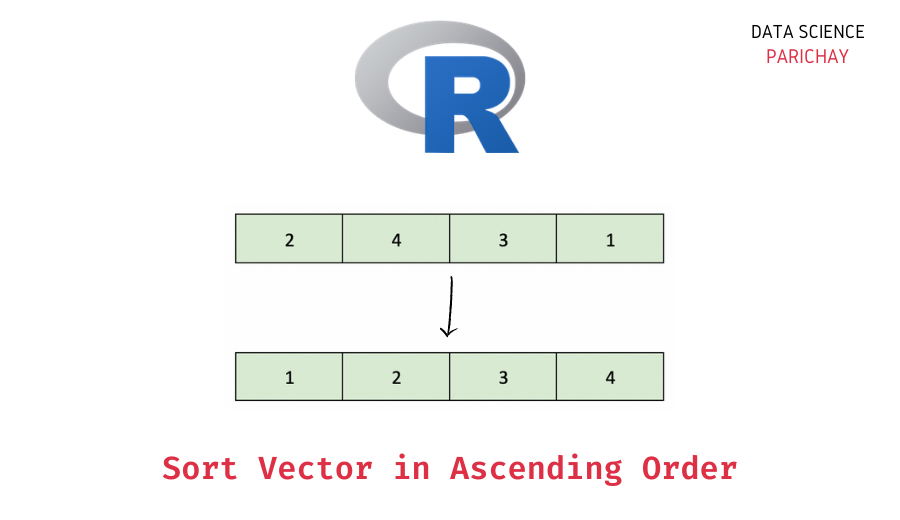# R – Sort a Vector in Ascending Order

In this tutorial, we will look at how to sort a vector in ascending order in the R programming language with the help of some examples.

## How do you sort a vector in R?

You can use the built-in `sort()` function to sort a vector in R. It sorts the vector in ascending order by default.

The following is the syntax –

`sort(x, decreasing=FALSE, na.last=NA)`

It returns the sorted vector.

The `sort()` function takes the following arguments –

• `x` – The object (in our case a vector) to sort.
• `decreasing` (optional) – Whether to sort `x` in descending order. It is `FALSE` by default.
• `na.last` (optional) – How to treat the `NA` values in `x`. If it’s `TRUE`, the `NA` values are put last, if it’s `FALSE`, the `NA` values are put first and if it’s `NA`, the `NA` values are removed in the sorted vector. It is `NA` by default.

## Steps to sort a vector in ascending order in R

Let’s now look at a step-by-step example of using the `sort()` function to sort a vector in ascending order.

### Step 1 – Create a vector

First, we will create a vector of some numbers that we will be using throughout this tutorial.

```# create a vector
vec <- c(4, 6, 2, 1, 3, 7, 5)
# display the vector
print(vec)```

Output:

📚 Data Science Programs By Skill Level

Introductory

Intermediate ⭐⭐⭐

🔎 Find Data Science Programs 👨‍💻 111,889 already enrolled

Disclaimer: Data Science Parichay is reader supported. When you purchase a course through a link on this site, we may earn a small commission at no additional cost to you. Earned commissions help support this website and its team of writers.

` 4 6 2 1 3 7 5`

Here we created a vector, `vec` containing some numbers. You can see that the values in `vec` are not sorted.

### Step 2 – Sort the vector in ascending order

To sort the vector in ascending order, pass it to the `sort()` function.

```# sort vector
new_vec <- sort(vec)
# display the sorted vector
print(new_vec)```

Output:

` 1 2 3 4 5 6 7`

Here, we used the default arguments for the `sort()` function. You can see that the resulting vector is sorted in ascending order.

### Extra – Sort vector with `NA` values in ascending order

What if we sort a vector containing `NA` values in R?

Let’s find out.

```# create a vector
vec <- c(4, 6, 2, NA, 1, 3, NA, NA, 7, 5)
# sort vector
new_vec <- sort(vec)
# display the sorted vector
print(new_vec)```

Output:

` 1 2 3 4 5 6 7`

Here, we create a vector with some numbers and `NA` values and then sort it in ascending order using the `sort()` function. You can see that, by default, the `sort()` function removed the `NA` values and returned the sorted vector.

You can change this behavior. For example, if you don’t want to remove the `NA` values and want them sorted to the end, pass `na.last = TRUE` to the `sort()` function.

```# create a vector
vec <- c(4, 6, 2, NA, 1, 3, NA, NA, 7, 5)
# sort vector with NA to end
new_vec <- sort(vec, na.last = TRUE)
# display the sorted vector
print(new_vec)```

Output:

`  1  2  3  4  5  6  7 NA NA NA`

The `NA` values are sorted to the end.

If you want to keep the `NA` values at the beginning of the sorted vector, pass `na.last = FALSE`.

```# create a vector
vec <- c(4, 6, 2, NA, 1, 3, NA, NA, 7, 5)
# sort vector with NA to start
new_vec <- sort(vec, na.last = FALSE)
# display the sorted vector
print(new_vec)```

Output:

` NA NA NA  1  2  3  4  5  6  7`

The `NA` values are at the beginning of the sorted vector.

## Summary – Sort Vector in Ascending Order in R

In this tutorial, we looked at how to sort a vector in ascending order in R. The following is a short summary of the steps mentioned in this tutorial.

1. Create a vector (skip this step if you already have a vector to sort).
2. Use the `sort()` function to sort the vector in ascending order. It removes the `NA` values in the vector by default. To sort the `NA` values to the end, pass `na.last = TRUE`. To sort the `NA` values to the start, pass `na.last = FALSE`.

You might also be interested in –

•